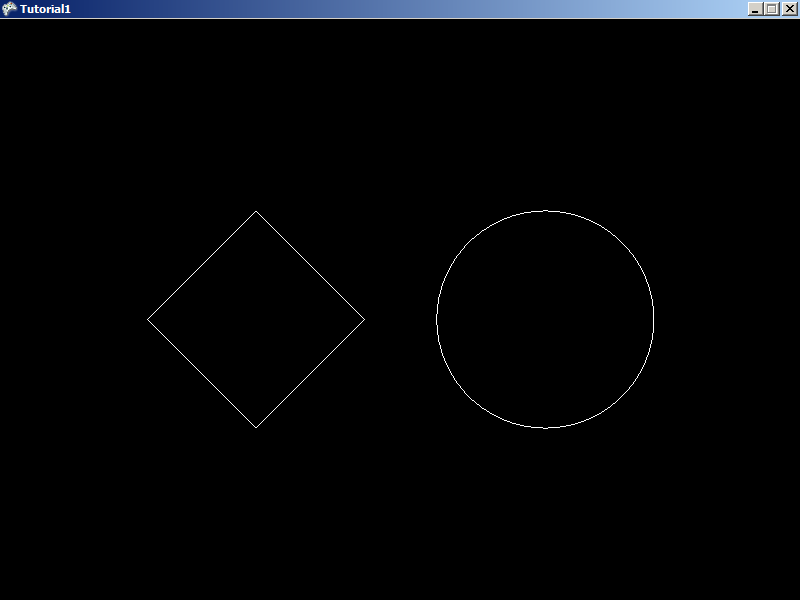# FlatRedBall.Math.Geometry.Polygon.CreateEquilateral

## Introduction

The CreateEquilateral static method is a method in the Polygon class which can be used to quickly create equilateral (same-length sides) Polygons.

## Code Example

The following code creates two Polygons. One is a 4-sided polygon. The other one is a very high-vertex count Polygon which can be used to draw smooth Circles. Keep in mind that high-vertex polygons like the one created in this example can be expensive when performing collisions.

```using FlatRedBall.Math.Geometry;
```

Add the following to Initialize after initializing FlatRedBall:

``` int numberOfSides = 4;
float firstAngle = 0;

Polygon firstPoly = Polygon.CreateEquilateral(numberOfSides, radius, firstAngle);
firstPoly.Visible = true;
firstPoly.X = -8;

// Increase the number of sides:
numberOfSides = 60;

// Now let's make a high-resolution Circle:
Polygon secondPolygon = Polygon.CreateEquilateral(numberOfSides, radius, firstAngle);
secondPolygon.Visible = true;
secondPolygon.X = 8;
```